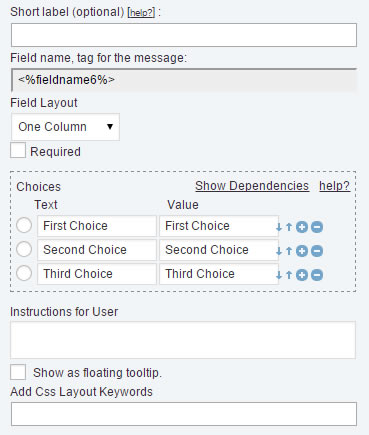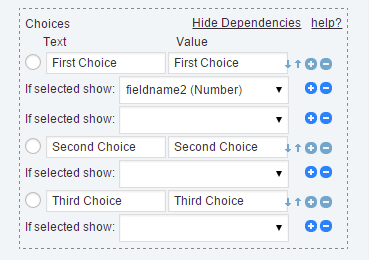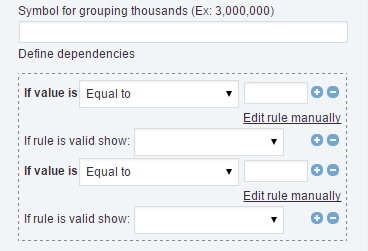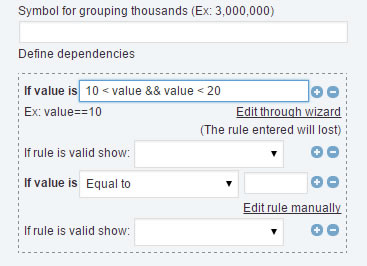# How to use dependencies between fields in the form?

The term of dependency is applied when a field depend of value in another field. The “Calculated Fields Form”, includes four controls that accept dependencies: The Checkbox control, the Radio control, the DropDown controls, and the Calculated Fields.

In the case of controls: DropDown, Checkbox, and Radio, the dependencies are created based in the option ticked from the list of choices. For the Calculated Fields the dependent fields are based in the value of calculated field as result of evaluate the equation.

The process in the controls: DropDown, Checkbox and Radio, is similar:

• Select the field in the form.
• Each of these fields includes a list of choices. Click the “Show dependencies link” into the choices section (fig.1)• The action displays a list of fields below each choice. Simply, select from the list the field to display when the choice is selected. If the choice is unticked, the dependent fields are set invisible, and its values will not taken into consideration in the equations, or submitted with the form.
• Beside the list of fields there are two buttons: +/- to add a new dependent field, or remove existing one(fig.2)The case of Calculated Field is singular. By general there is no way to know exactly the value returned by an equation, because it is determined by the values the user enter in the fields that participate in the equation. So, the process is more complex, but powerful.

• Select the calculated field in the form, and go to the “Define dependencies” section (fig.3)
• Each dependency is determined by a condition labeled “If the value is”, with a list of conditions for comparing (Equal to, Not equal to, Greater than, Greater than or equal to, Less than, Less than or equal to). The dependency includes a value, used as reference for comparing, and at least a dependent field, selected from the list labeled “If the is valid, show”In the case of calculated field there is a pair of buttons +/-, beside each dependency rule, and another pair of buttons +/-, beside each dependent field. The first pair of buttons allows create new dependency rules or remove existing one. The second pair of buttons allow add a new field to a dependency, or remove existing one.

But, how to define more specific comparison rules? How to display a third field, if the value of calculated field is greater than a number, but lesser than another?

The process require some additional skills but is not very hard:

Click the link: “Edit rule manually”, associated to the dependency rule, and type the validation rule manually, where the term “value” is a variable that represent the value of the calculated field.

For example, suppose you want display a field if the value of calculated field is greater than 10 but less than 20. For this case, click  the link: “Edit rule manually”, associated to the dependency rule, and type the following conditional statement: 10<value && value<20http://wordpress.dwbooster.com/forms/calculated-fields-form